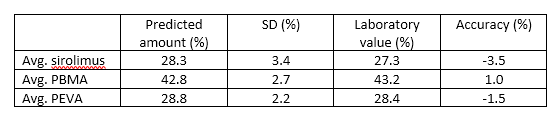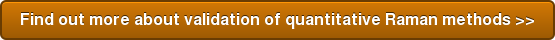# Spectroscopy Solutions Blog

Frederick H. Long from Spectroscopic Solutions, LLC, New Jersey, USA discusses quantitative prediction using Raman spectroscopy. Method validation is the process of verifying an analytical method is performing as required. It is required in regulated industries and is good scientific practice. United States Pharmacopeia chapters on Near IR spectroscopy and on Raman spectroscopy describe the criteria that should be used to validate a quantitative method using NIR or Raman spectroscopy. The criteria are accuracy, precision, linearity, range, robustness and specificity.Accuracy describes how close the predicted values are to the actual laboratory reference values. Precision defines how reproducible predictions are either in consecutive measurements or when different analysts perform the measurements. In validation of any chemometric method, the use of independent samples is essential. Testing with independent samples eliminates the possibility of overfitting the calibration set data. Furthermore the independent samples should challenge the model in question by presenting real-world variation to the model. The accuracy of the PLS models for sirolimus, PEVA and PBMA were tested with a set of independent production samples. The results are shown in Table 1.

Results and Discussion
Accuracy as expressed as a percent different between the lab and the PLS prediction values was less than 4% for all three components. The standard deviation (SD) values in Table 1 are a measure of the precision of the drug and polymer PLS methods.  We note that the PLS models were found to be more accurate than the simpler univariate models. This was even true in the cases of sirolimus, where a very strong peak associated with drug could be used for the univariate calibration. The sum of all predictions for the drug, PEVA, and PBMA ranged from 99.1 to 100.8%. This result demonstrates that PLS methods also exhibited mass balance.

Summary
In summary this article discusses the validation of PLS methods for drug, PEVA, and PBMA as determined by Raman spectroscopy. The validation of any method is essential in order to understand the performance of the method.Back to list